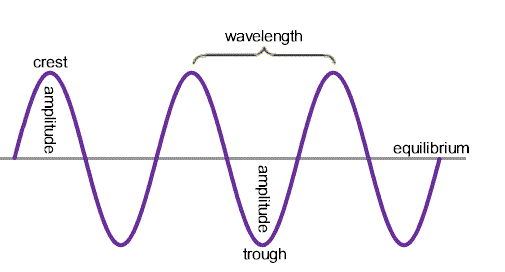# Propagation of Transverse Waves

Mechanical model for wave propagation comprises a row of spherical balls of equal masses, evenly spaced and connected together by identical springs. Imagine that by means of suitable device, ball-1, from left, is made to execute S.H.M. in a direction perpendicular to the row of balls with a period T. All the balls, owing to inertia of rest will not begin to oscillate at the same time. The motion is passed on from one ball to the next one by one.

Suppose that the time taken by the disturbance to travel from one ball to the next is T/8 s. This means that in the interval T/8 s, the disturbance propagates from the particle at mark 1 to the particle at mark 2. Similarly, in the next T/8 interval, the disturbance travels from the particle at mark 2 to the particle at mark 3 and so on.

At t = 0, all the particles are at their respective mean positions. At t = T, the first, fifth and ninth particles are at their respective mean positions. The first and ninth particles are about to move upward whereas the fifth particle is about to move downward. The third and seventh particles are at position of maximum displacement but on opposite sides of the horizontal axis.

The positions of third and seventh particles denote a trough and a crest, respectively.While the wave moves along the string, all particles of the string are oscillating up and down about their respective equilibrium positions with the same period (T) and amplitude (A). This wave remains progressive till it reaches the fixed end.

In a wave motion, the distance between the two nearest particles vibrating in the same phase is called a wavelength. It is denoted by λ.

Time taken by the wave to travel a distance λ is T. Therefore, the velocity of the wave is

v = Distance / Time

v = λ/T

But, 1/T= f, the frequency of the wave. Therefore,

v = fλ

If two consecutive particles in same state of motion are separated by a distance λ, the phase difference between them is 2π. Therefore, the phase change per unit distance

k = 2π/λ

k is the propagation constant. ω denotes phase change per unit time. But the phase change in time T is 2π, hence

ω = 2π/T = 2πf

Wave velocity is

v = ω/k = fλ